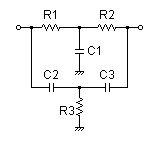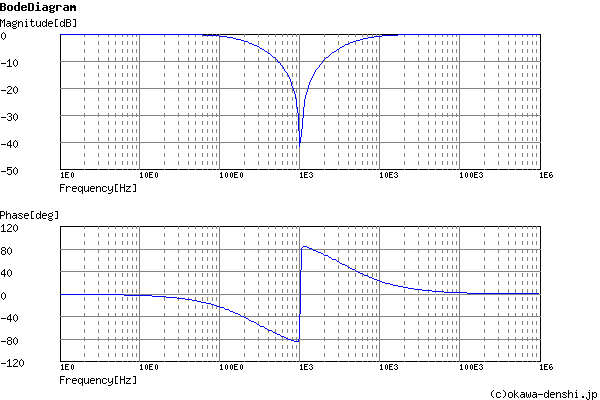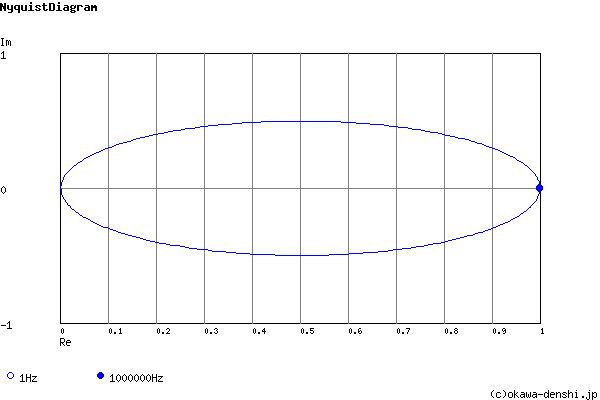# (Sample)Twin-T Notch Filter Design Tool - Result -

Calculated the transfer function for the Twin-T notch filter, displayed on graphs, showing Bode diagram, Nyquist diagram, Impulse response and Step response.

 Vin(s)→→Vout(s)
(Sample)Transfer Function:
 G(s)= s3+9090.90909091s2+41322314.0496s+368949232586 s3+36038.961039s2+284828807.556s+368949232586

R1 = 22kΩ
R2 = 22kΩ
R3 = 5.6kΩ
C1 = 0.01uF
C2 = 0.01uF
C3 = 0.01uF

#### Center rejection frequency

f0 = 1023.08672291[Hz]
f0 = 1013.91087188[Hz]

#### Pole(s)

p = -255.895392582[Hz]
|p|= 255.895392582[Hz]
p = -4041.77097335[Hz]
|p|= 4041.77097335[Hz]
p = -1438.11242732[Hz]
|p|= 1438.11242732[Hz]

#### Zero(s)

z = -4.34076355049 +1016.95722029i[Hz]
|z|= 1016.96648427[Hz]
z = -1438.18159192[Hz]
|z|= 1438.18159192[Hz]
z = -4.34076355049-1016.95722029i[Hz]
|z|= 1016.96648427[Hz]

#### Phase margin

pm= NAN[deg] (f =0[Hz])

#### Overshoot (in absolute value)

The 1st peak  gpk = 0.12 (t =0.00012[sec])

#### Final value of the step response (on the condition that the system converged when t goes to infinity)

g(∞) = 1

f0=Hz
 C1 = F C2 = F C3 = F
C1, C2, C3 is optional. But when setting these capacitances, C1, C2 and C3 of all are needed to give.

Select Capacitor Sequence:
Select Resistor Sequence:

### Frequency analysis

Bode diagram
Phase  Group delay
Nyquist diagram
Pole, zero
Phase margin
Oscillation analysis
Analysis on frequency range:
f1=∼f2=[Hz] (optional)

### Transient analysis

Step response
Impulse response
Overshoot
Final value of the step response
Analysis on time range:
0∼[sec] (optional)

# Frequency analysis# Transient analysis